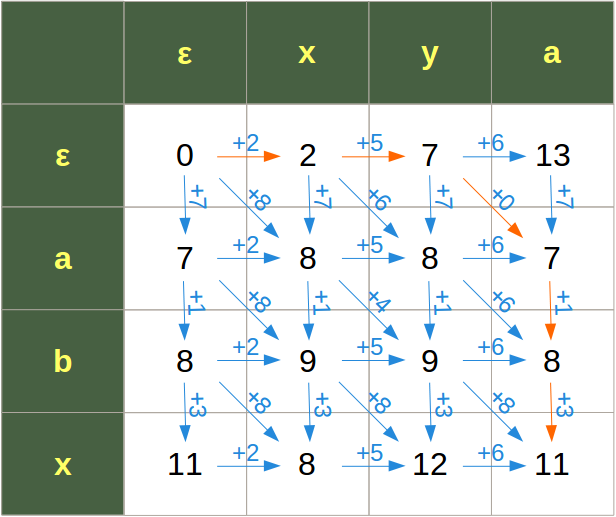## Levenshtein Distance

### Introduction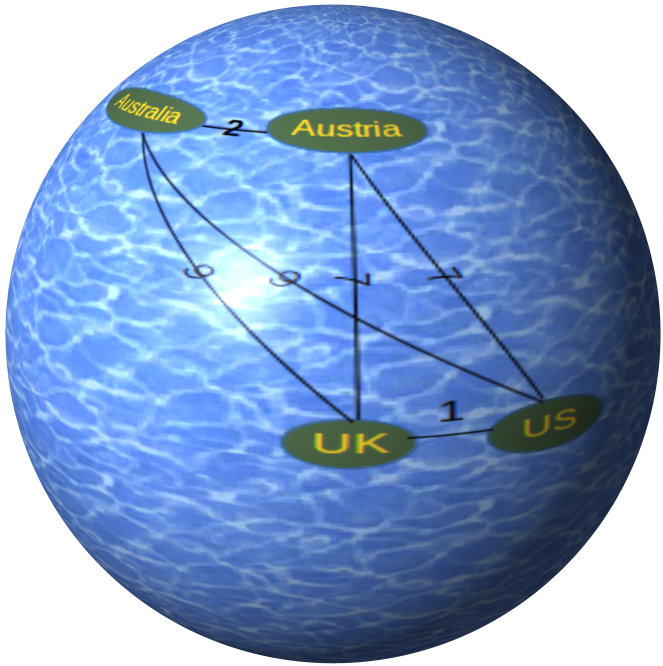This chapter covers the Levenshtein distance and presents some Python implementations for this measure. There are lots of use cases for the Levenshtein distances. The Levenshtein Distance and the underlying ideas are widely used in areas like computer science, computer linguistics, and even bioinformatics, molecular biology, DNA analysis. You can even measure the similarity of melodies or rhythms in music1. The Levenshtein distance has widely permeated our everyday life. Whenever you use a program or an application using some form of spell checking and error correction, the programmers most likely will have used "edit distance" or as it is also called "Levenshtein distance".

You might already have encountered another possible use case for this concept: Imagine that you are using a Python dictionary, in which you use strings as keys.

Let us look at the following example dictionary with city names of the United States, which are often misspelled:

cities = {"Pittsburgh":"Pennsylvania",
"Tucson":"Arizona",
"Cincinnati":"Ohio",
"Albuquerque":"New Mexico",
"Culpeper":"Virginia",
"Asheville":"North Carolina",
"Worcester":"Massachusetts",
"Manhattan":"New York",
"Phoenix":"Arizona",
"Niagara Falls":"New York"}


So, trying to get the corresponding state names via the following dictionary accesses will raise exceptions:

 cities["Tuscon"] cities["Pittsburg"] cities["Cincinati"] cities["Albequerque"] 

If a human reader looks at these misspellings, he or she will have no problem in recognizing the city you have in mind. The Python dictionary on the other hand is pedantic and unforgivable. It only accepts a key, if it is exactly identical.

The question is to what degree are two strings similar? What we need is a string similarity metric or a measure for the "distance" of strings.

A string metric is a metric that measures the distance between two text strings. One of the best known string metrics is the so-called Levenshtein Distance, also known as Edit Distance. Levenshtein calculates the the number of substitutions and deletions needed in order to transform one string into another one.

### The Minimum Edit Distance or Levenshtein Dinstance

The minimum edit distance between two strings is the minimum numer of editing operations needed to convert one string into another. The editing operations can consist of insertions, deletions and substitutions.

The simplest sets of edit operations can be defined as:

• Insertion of a single symbol. This means that we add a character to a string s. Example: If we have the string s = "Manhatan", we can insert the character "t" to get the correct spelling:

>>> s = "Manhatan"
>>> s = s[:5] + "t" + s[5:]
>>> print(s)
Manhattan

• Deletion of a single symbol Example:

>>> s = "Mannhattan"
>>> s = s[:2] + s[3:]
>>> s
'Manhattan'

• Substitution of a single symbol In the following example, we have to change the letter "o" into the letter "a" to get the correct spelling:
>>> s = "Manhatton"
>>> s = s[:7] + "a" + s[8:]
>>> s
'Manhattan'


The minimum edit distance between the two strings "Mannhaton" and "Manhattan" corresponds to the value 3, as we need three basic editing operation to transform the first one into the second one:

>>> s = "Mannhaton"
>>> s = s[:2] + s[3:]         # deletion
>>> s
'Manhaton'
>>> s = s[:5] + "t" + s[5:]   # insertion
>>> s
'Manhatton'
>>> s = s[:7] + "a" + s[8:]   # substitution
>>> s
'Manhattan'


We can assign assign a weight or costs to each of these edit operations, e.g. setting each of them to 1. It is also possible to argue that substitutions should be more expensive than insertations or deletions, so sometimes the costs for substitutions are set to 2.

### Mathematical Definition of the Levenshtein Distance

The Levenshtein distance between two strings a and b is given by leva,b(len(a), len(b)) where leva,b(i, j) is equal to

• max(i, j) if min(i, j)=0
• otherwise:
min(leva,b(i-1, j) + 1,
leva,b(i, j-1) + 1,
leva,b(i-1, j-1) + 1ai≠bj)


where 1ai≠bj</sub> is the indicator function equal to 0 when ai=bj and equal to 1 otherwise, and leva,b(i, j) is the distance between the first i characters of a and the first j characters of b.

The Levenshtein distance has the following properties:

• It is zero if and only if the strings are equal.
• It is at least the difference of the sizes of the two strings.
• It is at most the length of the longer string.
• Triangle inequality: The Levenshtein distance between two strings is no greater than the sum of their Levenshtein distances from a third string.

### Recursive Levenshtein Function in Python

The following Python function implements the Levenshtein distance in a recursive way:

def LD(s, t):
if s == "":
return len(t)
if t == "":
return len(s)
if s[-1] == t[-1]:
cost = 0
else:
cost = 1

res = min([LD(s[:-1], t)+1,
LD(s, t[:-1])+1,
LD(s[:-1], t[:-1]) + cost])

return res

print(LD("Python", "Peithen"))

3


This recursive implementation is very inefficient because it recomputes the Levenshtein distance of the same substrings over and over again. We count the number of calls in the following version by using a decorator function. If you don't know them, you can learn about them in our chapter on Memoization and Decorators:

from collections import Counter

def call_counter(func):
def helper(*args, **kwargs):
helper.calls += 1
key = str(args) + str(kwargs)
helper.c[key] += 1
return func(*args, **kwargs)
helper.c = Counter()
helper.calls = 0
helper.__name__= func.__name__

return helper

@call_counter
def LD(s, t):
if s == "":
return len(t)
if t == "":
return len(s)
if s[-1] == t[-1]:
cost = 0
else:
cost = 1

res = min([LD(s[:-1], t)+1,
LD(s, t[:-1])+1,
LD(s[:-1], t[:-1]) + cost])

return res

print(LD("Python", "Peithen"))

print("LD was called " + str(LD.calls) + " times!")
print(LD.c.most_common())

3
LD was called 29737 times!
[("('', 'P'){}", 5336), ("('P', ''){}", 4942), ("('', ''){}", 3653), ("('P', 'P'){}", 3653), ("('', 'Pe'){}", 2364), ("('P', 'Pe'){}", 1683), ("('Py', ''){}", 1666), ("('Py', 'P'){}", 1289), ("('', 'Pei'){}", 912), ("('Py', 'Pe'){}", 681), ("('P', 'Pei'){}", 681), ("('Pyt', ''){}", 462), ("('Pyt', 'P'){}", 377), ("('Py', 'Pei'){}", 321), ("('', 'Peit'){}", 292), ("('Pyt', 'Pe'){}", 231), ("('P', 'Peit'){}", 231), ("('Py', 'Peit'){}", 129), ("('Pyt', 'Pei'){}", 129), ("('Pyth', ''){}", 98), ("('Pyth', 'P'){}", 85), ("('', 'Peith'){}", 72), ("('Pyt', 'Peit'){}", 63), ("('Pyth', 'Pe'){}", 61), ("('P', 'Peith'){}", 61), ("('Py', 'Peith'){}", 41), ("('Pyth', 'Pei'){}", 41), ("('Pyth', 'Peit'){}", 25), ("('Pyt', 'Peith'){}", 25), ("('Pytho', ''){}", 14), ("('Pyth', 'Peith'){}", 13), ("('Pytho', 'P'){}", 13), ("('', 'Peithe'){}", 12), ("('Pytho', 'Pe'){}", 11), ("('P', 'Peithe'){}", 11), ("('Py', 'Peithe'){}", 9), ("('Pytho', 'Pei'){}", 9), ("('Pyt', 'Peithe'){}", 7), ("('Pytho', 'Peit'){}", 7), ("('Pyth', 'Peithe'){}", 5), ("('Pytho', 'Peith'){}", 5), ("('Pytho', 'Peithe'){}", 3), ("('Python', 'Pei'){}", 1), ("('Python', 'Peithe'){}", 1), ("('', 'Peithen'){}", 1), ("('P', 'Peithen'){}", 1), ("('Pytho', 'Peithen'){}", 1), ("('Py', 'Peithen'){}", 1), ("('Python', 'P'){}", 1), ("('Python', 'Peit'){}", 1), ("('Pyt', 'Peithen'){}", 1), ("('Pyth', 'Peithen'){}", 1), ("('Python', 'Peith'){}", 1), ("('Python', ''){}", 1), ("('Python', 'Pe'){}", 1), ("('Python', 'Peithen'){}", 1)]


We can see that this recursive function is highly inefficient. The Levenshtein distance of the string s="" and t="P" was calculated 5336 times. In the following version we add some "memory" to our recursive Levenshtein function by adding a dictionary memo:

def call_counter(func):
def helper(*args, **kwargs):
helper.calls += 1
return func(*args, **kwargs)
helper.calls = 0
helper.__name__= func.__name__

return helper

memo = {}
@call_counter
def levenshtein(s, t):
if s == "":
return len(t)
if t == "":
return len(s)
cost = 0 if s[-1] == t[-1] else 1

i1 = (s[:-1], t)
if not i1 in memo:
memo[i1] = levenshtein(*i1)
i2 = (s, t[:-1])
if not i2 in memo:
memo[i2] = levenshtein(*i2)
i3 = (s[:-1], t[:-1])
if not i3 in memo:
memo[i3] = levenshtein(*i3)
res = min([memo[i1]+1, memo[i2]+1, memo[i3]+cost])

return res

print(levenshtein("Python", "Pethno"))

print("The function was called " + str(levenshtein.calls) + " times!")

3
The function was called 49 times!


The previous recursive version is now efficient, but it has a design flaw in it. We polluted the code with the statements to update our dictionary mem. Of course, the design is a lot better, if we do not pollute our code by adding the logic for saving the values into our Levenshtein function. We can also "outsource" this code into a decorator. The following version uses a decorator "memoize" to save these values:

def call_counter(func):
def helper(*args, **kwargs):
helper.calls += 1
return func(*args, **kwargs)
helper.calls = 0
helper.__name__= func.__name__

return helper

def memoize(func):
mem = {}
def memoizer(*args, **kwargs):
key = str(args) + str(kwargs)
if key not in mem:
mem[key] = func(*args, **kwargs)
return mem[key]
return memoizer

@call_counter
@memoize
def levenshtein(s, t):
if s == "":
return len(t)
if t == "":
return len(s)
if s[-1] == t[-1]:
cost = 0
else:
cost = 1

res = min([levenshtein(s[:-1], t)+1,
levenshtein(s, t[:-1])+1,
levenshtein(s[:-1], t[:-1]) + cost])

return res

print(levenshtein("Python", "Peithen"))

print("The function was called " + str(levenshtein.calls) + " times!")

3
The function was called 127 times!


The additional calls come from the fact that we have three unconditional calls as arguments of the function "min".

### Iterative Computation of the Levenshtein Distance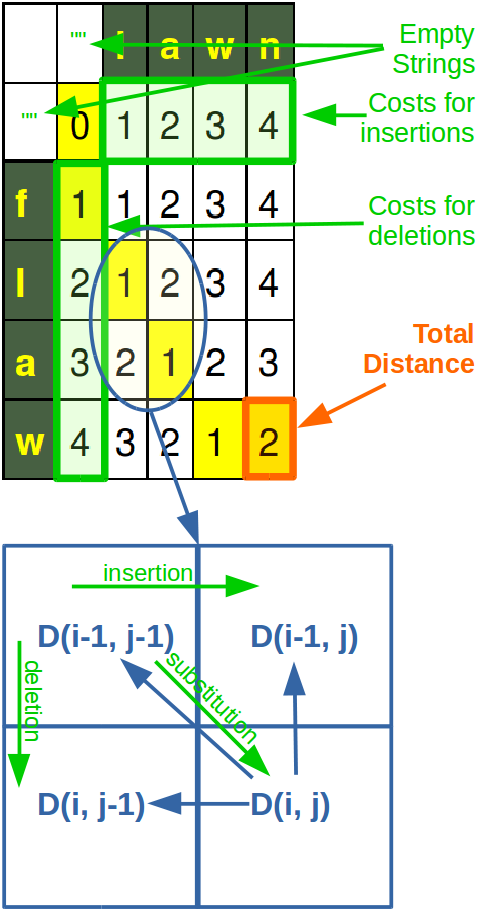To compute the Levenshtein distance in a non-recursive way, we use a matrix containing the Levenshtein distances between all prefixes of the first string and all prefixes of the second one. We can dynamically compute the values in this matrix. The last value computed will be the distance between the two full strings. This is an algorithmic example of a bottom-up dynamic programming.

The algorithm works like this:
We set the cost for an insertion, a deletion and a substitution to 1. We want to calculate the distance between two string s and t with len(s) == m and len(t) == n. A matrix D is used, which contains in the (i,j)-cell the Levenshtein distance between s[:i+1] and t[:j+1]. The values of the matrix will be calculated starting with the upper left corner and ending with the lower right corner.

We start with filling in the base cases, i.e. the row and the column with the index 0. Calculation in this case means that we fill the row with index 0 with the lenghts of the substrings of t and respectively fill the column with the index 0 with the lengths of the substrings of s.

The values of all the other elements of the matrix only depend on the values of their left neighbour, the top neightbour and the top left one.

The calculation of the D(i,j) for both i and j greater 0 works like this: D(i,j) means that we are calculating the Levenshtein distance of the substrings s[0:i-1] and t[0:j-1]. If the last characters of these substrings are equal, the edit distance corresponds to the distance of the substrings s[0:-1] and t[0:-1], which may be empty, if s or t consists of only one character, which means that we will use the values from the 0th column or row. If the last characters of s[0:i-1] and t[0:j-1] are not equal, the edit distance D[i,j] will be set to the sum of 1 + min(D[i, j-1], D[i-1, j], D[i-1, j-1])-

We illustrate this in the following diagram:

def iterative_levenshtein(s, t):
"""
iterative_levenshtein(s, t) -> ldist
ldist is the Levenshtein distance between the strings
s and t.
For all i and j, dist[i,j] will contain the Levenshtein
distance between the first i characters of s and the
first j characters of t
"""

rows = len(s)+1
cols = len(t)+1
dist = [[0 for x in range(cols)] for x in range(rows)]

# source prefixes can be transformed into empty strings
# by deletions:
for i in range(1, rows):
dist[i] = i

# target prefixes can be created from an empty source string
# by inserting the characters
for i in range(1, cols):
dist[i] = i

for col in range(1, cols):
for row in range(1, rows):
if s[row-1] == t[col-1]:
cost = 0
else:
cost = 1
dist[row][col] = min(dist[row-1][col] + 1,      # deletion
dist[row][col-1] + 1,      # insertion
dist[row-1][col-1] + cost) # substitution

for r in range(rows):
print(dist[r])

return dist[row][col]

print(iterative_levenshtein("flaw", "lawn"))

[0, 1, 2, 3, 4]
[1, 1, 2, 3, 4]
[2, 1, 2, 3, 4]
[3, 2, 1, 2, 3]
[4, 3, 2, 1, 2]
2


The following picture of the matrix of our previous calculation contains - coloured in yellow - the optimal path through the matrix. We start with a deletion ("f"), we keep the "l" (no costs added), after this we keep the "a" and "w". The last step is an insertion, raising the costs to 2, which is the final Levenstein distance.

For the sake of another example, let us use the Levenshtein distance for our initial example of this chapter. So, we will virtually "go back" to New York City and its thrilling borough Manhattan. We compare it with a misspelling "Manahaton", which is the combination of various common misspellings.

print(iterative_levenshtein("Manhattan", "Manahaton"))

[0, 1, 2, 3, 4, 5, 6, 7, 8, 9]
[1, 0, 1, 2, 3, 4, 5, 6, 7, 8]
[2, 1, 0, 1, 2, 3, 4, 5, 6, 7]
[3, 2, 1, 0, 1, 2, 3, 4, 5, 6]
[4, 3, 2, 1, 1, 1, 2, 3, 4, 5]
[5, 4, 3, 2, 1, 2, 1, 2, 3, 4]
[6, 5, 4, 3, 2, 2, 2, 1, 2, 3]
[7, 6, 5, 4, 3, 3, 3, 2, 2, 3]
[8, 7, 6, 5, 4, 4, 3, 3, 3, 3]
[9, 8, 7, 6, 5, 5, 4, 4, 4, 3]
3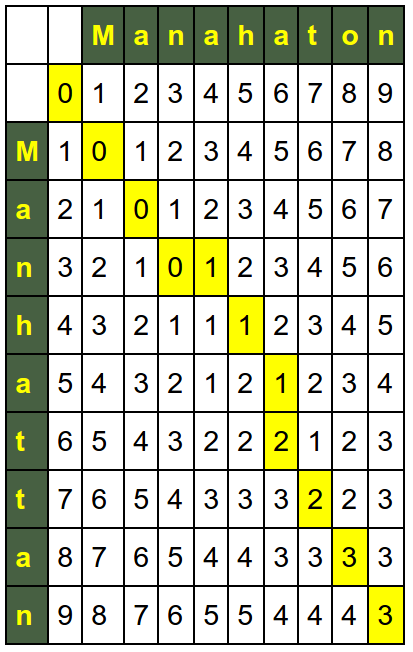So far we have had fixed costs for insertions, deletions and substitutions, i.e. each of them was set to 1.

def iterative_levenshtein(s, t, costs=(1, 1, 1)):
"""
iterative_levenshtein(s, t) -> ldist
ldist is the Levenshtein distance between the strings
s and t.
For all i and j, dist[i,j] will contain the Levenshtein
distance between the first i characters of s and the
first j characters of t

costs: a tuple or a list with three integers (d, i, s)
where d defines the costs for a deletion
i defines the costs for an insertion and
s defines the costs for a substitution
"""

rows = len(s)+1
cols = len(t)+1
deletes, inserts, substitutes = costs

dist = [[0 for x in range(cols)] for x in range(rows)]

# source prefixes can be transformed into empty strings
# by deletions:
for row in range(1, rows):
dist[row] = row * deletes

# target prefixes can be created from an empty source string
# by inserting the characters
for col in range(1, cols):
dist[col] = col * inserts

for col in range(1, cols):
for row in range(1, rows):
if s[row-1] == t[col-1]:
cost = 0
else:
cost = substitutes
dist[row][col] = min(dist[row-1][col] + deletes,
dist[row][col-1] + inserts,
dist[row-1][col-1] + cost) # substitution

for r in range(rows):
print(dist[r])

return dist[row][col]

# default:
print(iterative_levenshtein("abc", "xyz"))
# the costs for substitions are twice as high as inserts and delets:
print(iterative_levenshtein("abc", "xyz", costs=(1, 1, 2)))
# inserts and deletes are twice as high as substitutes
print(iterative_levenshtein("abc", "xyz", costs=(2, 2, 1)))

[0, 1, 2, 3]
[1, 1, 2, 3]
[2, 2, 2, 3]
[3, 3, 3, 3]
3
[0, 1, 2, 3]
[1, 2, 3, 4]
[2, 3, 4, 5]
[3, 4, 5, 6]
6
[0, 2, 4, 6]
[2, 1, 3, 5]
[4, 3, 2, 4]
[6, 5, 4, 3]
3


The situation in the call to iterative_levenshtein with default costs, i.e. 1 for insertions, deletions and substitutions: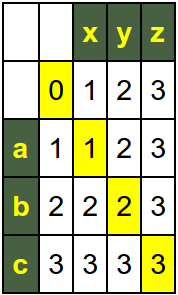The content of the matrix if we the substitutions are twice as expensive as the insertions and deletions, i.e. the call iterative_levenshtein("abc", "xyz", costs=(1, 1, 2)):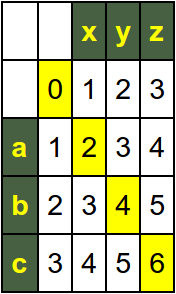Now we call iterative_levenshtein("abc", "xyz", costs=(2, 2, 1)), which means that a substitution is half as expension as an insertion or a deletion: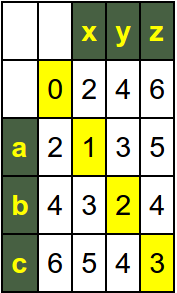It is also possible to have indivual weights for each character. Instead of passing a tuple with three values to the function, we will use a dictionary with values for every character.

def iterative_levenshtein(s, t, **weight_dict):
"""
iterative_levenshtein(s, t) -> ldist
ldist is the Levenshtein distance between the strings
s and t.
For all i and j, dist[i,j] will contain the Levenshtein
distance between the first i characters of s and the
first j characters of t

weight_dict: keyword parameters setting the costs for characters,
the default value for a character will be 1
"""

rows = len(s)+1
cols = len(t)+1

alphabet = "abcdefghijklmnopqrstuvwxyz"
w = dict( (x, (1, 1, 1)) for x in alphabet + alphabet.upper())
if weight_dict:
w.update(weight_dict)

dist = [[0 for x in range(cols)] for x in range(rows)]

# source prefixes can be transformed into empty strings
# by deletions:
for row in range(1, rows):
dist[row] = dist[row-1] + w[s[row-1]]

# target prefixes can be created from an empty source string
# by inserting the characters
for col in range(1, cols):
dist[col] = dist[col-1] + w[t[col-1]]

for col in range(1, cols):
for row in range(1, rows):
deletes = w[s[row-1]]
inserts = w[t[col-1]]
subs = max( (w[s[row-1]], w[t[col-1]]))
if s[row-1] == t[col-1]:
subs = 0
else:
subs = subs

dist[row][col] = min(dist[row-1][col] + deletes,
dist[row][col-1] + inserts,
dist[row-1][col-1] + subs) # substitution

for r in range(rows):
print(dist[r])

return dist[row][col]

# default:
print(iterative_levenshtein("abx",
"xya",
x=(3, 2, 8),
y=(4, 5, 4),
a=(7, 6, 6)) )
#print(iterative_levenshtein("abc", "xyz", costs=(1,1,substitution_costs)))

subs:  8 deletes:  7 inserts:  2 a x
subs:  8 deletes:  1 inserts:  2 b x
subs:  0 deletes:  3 inserts:  2 x x
subs:  6 deletes:  7 inserts:  5 a y
subs:  4 deletes:  1 inserts:  5 b y
subs:  8 deletes:  3 inserts:  5 x y
subs:  0 deletes:  7 inserts:  6 a a
subs:  6 deletes:  1 inserts:  6 b a
subs:  8 deletes:  3 inserts:  6 x a
[0, 2, 7, 13]
[7, 8, 8, 7]
[8, 9, 9, 8]
[11, 8, 12, 11]
11


We demonstrate in the following diagram how the algorithm works with the weighted characters. The orange arrows show the path to the minimal edit distance 11: